### IMO Shortlist 1974 problem 10

Kvaliteta:
Avg: 0,0
Težina:
Avg: 0,0
Let$ABC$ be a triangle. Prove that there exists a point$D$ on the side$AB$ of the triangle$ABC$, such that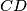$CD$ is the geometric mean of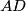$AD$ and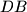$DB$, iff the triangle$ABC$ satisfies the inequality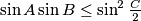$\sin A\sin B\le\sin^2\frac{C}{2}$.

CommentAlternative formulation, from IMO ShortList 1974, Finland 2: We consider a triangle$ABC$. Prove that: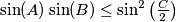$\sin(A) \sin(B) \leq \sin^2 \left( \frac{C}{2} \right)$ is a necessary and sufficient condition for the existence of a point$D$ on the segment$AB$ so that$CD$ is the geometrical mean of$AD$ and$BD$.
Izvor: Međunarodna matematička olimpijada, shortlist 1974